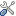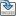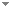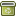# Brouwer’s Fan Theorem as an axiom and as a contrast to Kleene’s alternative

Archive for Mathematical Logic 53 (5-6):621-693 (2014)

 Abstract The paper is a contribution to intuitionistic reverse mathematics. We introduce a formal system called Basic Intuitionistic MathematicsBIM, and then search for statements that are, over BIM, equivalent to Brouwer’s Fan Theorem or to its positive denial, Kleene’s Alternative to the Fan Theorem. The Fan Theorem is true under the intended intuitionistic interpretation and Kleene’s Alternative is true in the model of BIM consisting of the Turing-computable functions. The task of finding equivalents of Kleene’s Alternative is, intuitionistically, a nontrivial extension of the task of finding equivalents of the Fan Theorem, although there is a certain symmetry in the arguments that we shall try to make transparent. We introduce closed-and-separable subsets of Baire space N\documentclass[12pt]{minimal} \usepackage{amsmath} \usepackage{wasysym} \usepackage{amsfonts} \usepackage{amssymb} \usepackage{amsbsy} \usepackage{mathrsfs} \usepackage{upgreek} \setlength{\oddsidemargin}{-69pt} \begin{document}$${\mathcal{N}}$$\end{document} and of the set R\documentclass[12pt]{minimal} \usepackage{amsmath} \usepackage{wasysym} \usepackage{amsfonts} \usepackage{amssymb} \usepackage{amsbsy} \usepackage{mathrsfs} \usepackage{upgreek} \setlength{\oddsidemargin}{-69pt} \begin{document}$${\mathcal{R}}$$\end{document} of the real numbers. Such sets may be compact and also positively noncompact. The Fan Theorem is the statement that Cantor space C\documentclass[12pt]{minimal} \usepackage{amsmath} \usepackage{wasysym} \usepackage{amsfonts} \usepackage{amssymb} \usepackage{amsbsy} \usepackage{mathrsfs} \usepackage{upgreek} \setlength{\oddsidemargin}{-69pt} \begin{document}$${\mathcal{C}}$$\end{document}, or, equivalently, the unit interval [0, 1], is compact and Kleene’s Alternative is the statement that C\documentclass[12pt]{minimal} \usepackage{amsmath} \usepackage{wasysym} \usepackage{amsfonts} \usepackage{amssymb} \usepackage{amsbsy} \usepackage{mathrsfs} \usepackage{upgreek} \setlength{\oddsidemargin}{-69pt} \begin{document}$${\mathcal{C}}$$\end{document}, or, equivalently, [0, 1], is positively noncompact. The class of the compact closed-and-separable sets and also the class of the closed-and-separable sets that are positively noncompact are characterized in many different ways and a host of equivalents of both the Fan Theorem and Kleene’s Alternative is found. Keywords No keywords specified (fix it) Categories (categorize this paper) ISBN(s) DOI 10.1007/s00153-014-0384-9 OptionsEdit this recordMark as duplicateExport citationFind it on ScholarRequest removal from indexRevision history

PhilArchive copy

Upload a copy of this paper     Check publisher's policy     Papers currently archived: 71,231

## References found in this work BETA

Foundations of Constructive Analysis.John Myhill - 1972 - Journal of Symbolic Logic 37 (4):744-747.
Foundations of Constructive Analysis.Errett Bishop - 1967 - New York, NY, USA: Mcgraw-Hill.
Equivalents of the (Weak) Fan Theorem.Iris Loeb - 2005 - Annals of Pure and Applied Logic 132 (1):51-66.

## Citations of this work BETA

Classifying Dini's Theorem.Josef Berger & Peter Schuster - 2006 - Notre Dame Journal of Formal Logic 47 (2):253-262.

## Similar books and articles

Brouwer's Equivalence Between Virtual and Inextensible Order.Enrico Martino - 1988 - History and Philosophy of Logic 9 (1):57-66.
Book Review: Mark van Atten. On Brouwer. [REVIEW]O. Bradley Bassler - 2006 - Notre Dame Journal of Formal Logic 47 (4):581-599.
Realizing Brouwer's Sequences.Richard E. Vesley - 1996 - Annals of Pure and Applied Logic 81 (1-3):25-74.
Alternative Proof of a Theorem of Kleene.Hao Wang - 1958 - Journal of Symbolic Logic 23 (3):250.
Reclassifying the Antithesis of Specker’s Theorem.Hannes Diener - 2012 - Archive for Mathematical Logic 51 (7-8):687-693.
Weak König's Lemma Implies Brouwer's Fan Theorem: A Direct Proof.Hajime Ishihara - 2006 - Notre Dame Journal of Formal Logic 47 (2):249-252.
Induktive Definitionen Und Dilatoren.Wilfried Buchholz - 1988 - Archive for Mathematical Logic 27 (1):51-60.
Satisfying Predicates: Kleene's Proof of the Hilbert–Bernays Theorem.Gary Ebbs - 2015 - History and Philosophy of Logic 36 (4):346-366.
The Fine Structure of the Intuitionistic Borel Hierarchy.Wim Veldman - 2009 - Review of Symbolic Logic 2 (1):30-101.
Local Density of Kleene Degrees.Hisato Muraki - 1995 - Mathematical Logic Quarterly 41 (2):183-189.